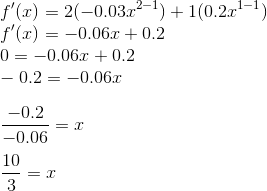Math Modeling : 5-Step Method

Example Questions

Example Question #1 : 5 Step Method

A cow that weighs 175 pounds gains 3 pounds per day, and it costs 30 cents per day to keep that cow. The market price for cows is 75 cents per pound, but the price is falling one cent per day. When should the cow be sold to make the maximum profit?

Use the 5-step method.

The cow should be sold after 4 days.

The cow should be sold after 2 days.

The max profit is $131.58. The cow should be sold after 3 days. The max profit is$151.58.

The cow should be sold after 3 days.

Explanation:

The 5-step method for this particular modeling question is as follows.

When should the cow be sold to make the maximum profit?

Now identify all known information.

Variables: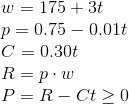2. Select the modeling approach

For this particular question the function can be define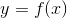on a subset of the real number line. Therefore, take the derivative ofand set it equal to zero to calculate the maximum.

3. Formulate the model

To maximizeformulate the equation in terms of.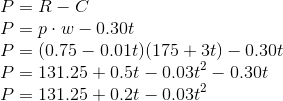Now rewrite the equation in terms of.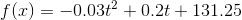4. Solve the model

Now to solve the model, take the derivative, set it equal to zero, and solve for.